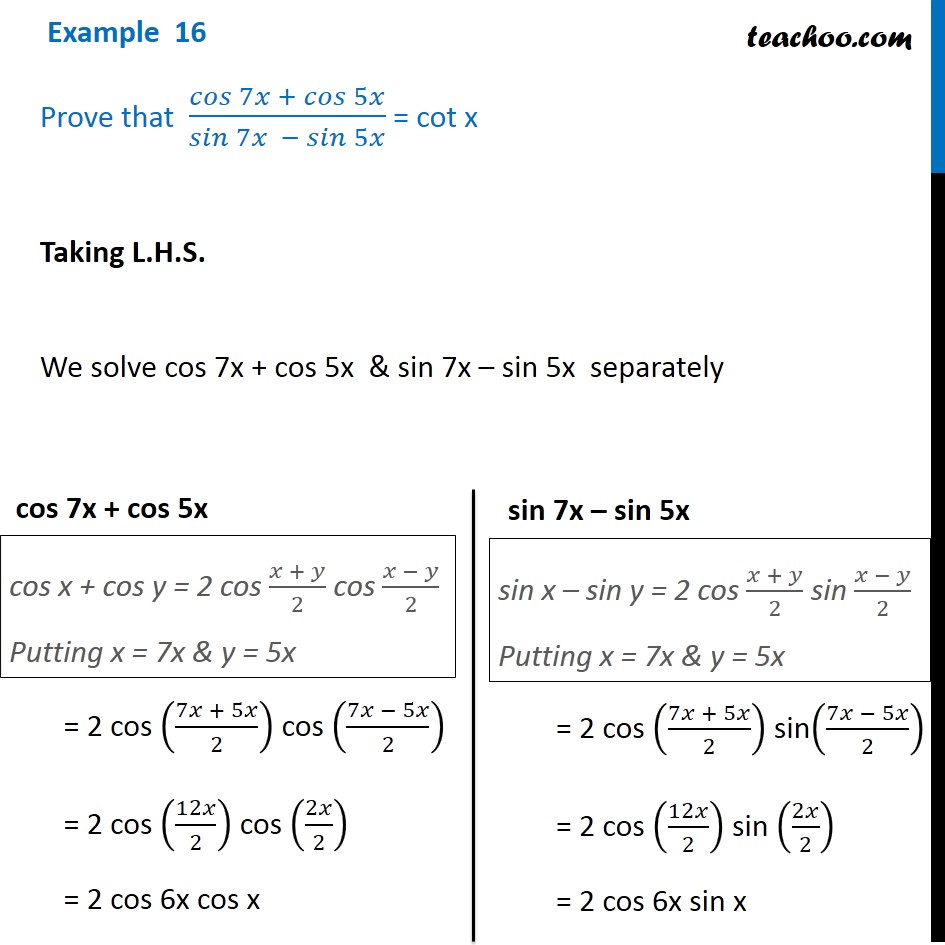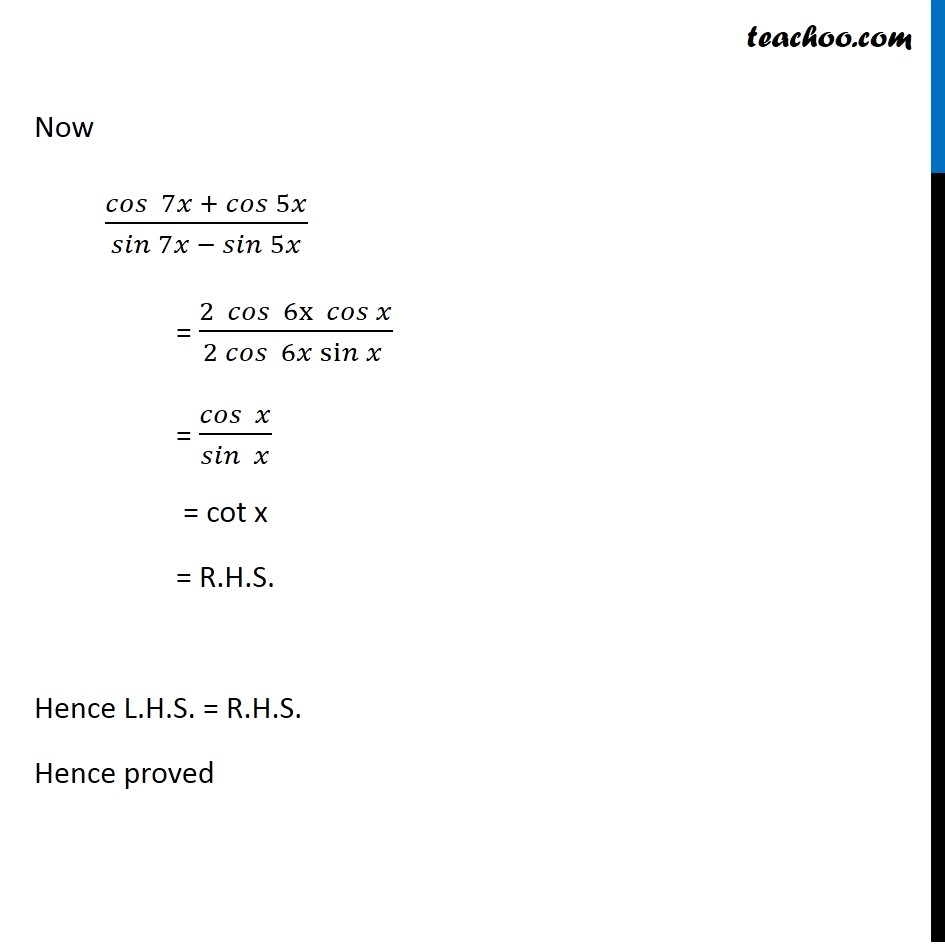Subscribe to our Youtube Channel - https://you.tube/teachoo

1. Chapter 3 Class 11 Trigonometric Functions
2. Concept wise
3. Cos x + cos y formula

Transcript

Example 16 Prove that 𝑐𝑜𝑠⁡〖7𝑥 + 𝑐𝑜𝑠⁡5𝑥 〗/𝑠𝑖𝑛⁡〖7𝑥 − 𝑠𝑖𝑛⁡5𝑥 〗 = cot x Taking L.H.S. We solve cos 7x + cos 5x & sin 7x – sin 5x separately cos x + cos y = 2 cos (𝑥 + 𝑦)/2 cos (𝑥 − 𝑦)/2 Putting x = 7x & y = 5x cos 7x + cos 5x = 2 cos ((7𝑥 + 5𝑥)/2) cos ((7𝑥 − 5𝑥)/2) = 2 cos (12𝑥/2) cos (2𝑥/2) = 2 cos 6x cos x sin x – sin y = 2 cos (𝑥 + 𝑦)/2 sin (𝑥 − 𝑦)/2 Putting x = 7x & y = 5x sin 7x – sin 5x = 2 cos ((7𝑥 + 5𝑥)/2) sin((7𝑥 − 5𝑥)/2) = 2 cos (12𝑥/2) sin (2𝑥/2) = 2 cos 6x sin x Now 〖𝑐𝑜𝑠 〗⁡〖7𝑥 + 𝑐𝑜𝑠⁡5𝑥 〗/𝑠𝑖𝑛⁡〖7𝑥 − 𝑠𝑖𝑛⁡5𝑥 〗 = (2 〖 𝑐𝑜𝑠 〗⁡〖6x 𝑐𝑜𝑠⁡𝑥 〗)/(2 𝑐𝑜𝑠⁡〖 6𝑥 si𝑛⁡𝑥 〗 ) = 𝑐𝑜𝑠⁡〖 𝑥〗/𝑠𝑖𝑛⁡〖 𝑥〗 = cot x = R.H.S. Hence L.H.S. = R.H.S. Hence proved

Cos x + cos y formula